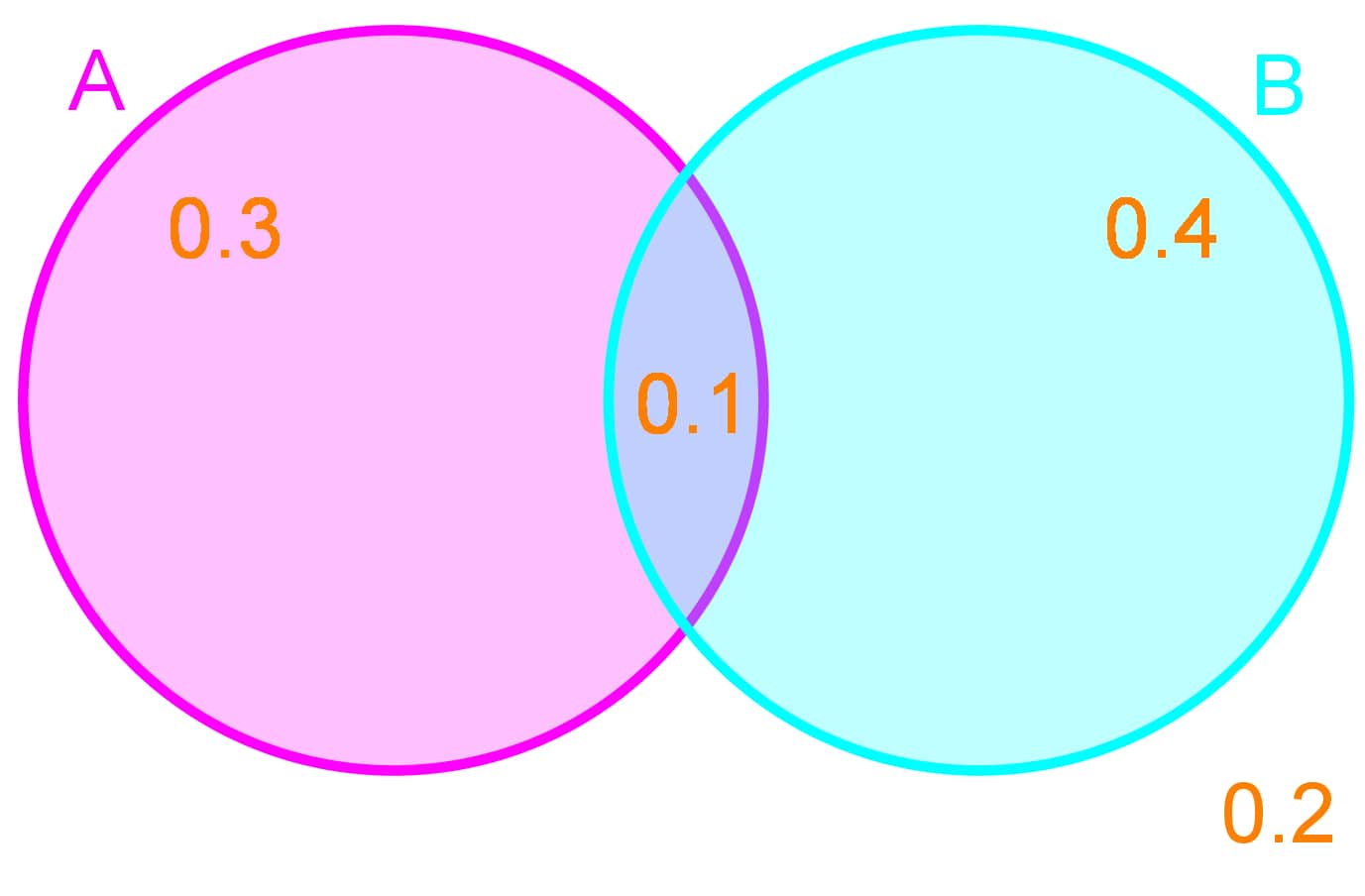# Probability with Venn diagrams - Probability

### Probability with Venn diagrams

#### Lessons

##### Determining the Complement of Events The probabilities of certain events are given in the Venn Diagram below: